Courses

# Test: Nuclear Power Plant - 1

## 20 Questions MCQ Test RRB JE for Mechanical Engineering | Test: Nuclear Power Plant - 1

Description
This mock test of Test: Nuclear Power Plant - 1 for Mechanical Engineering helps you for every Mechanical Engineering entrance exam. This contains 20 Multiple Choice Questions for Mechanical Engineering Test: Nuclear Power Plant - 1 (mcq) to study with solutions a complete question bank. The solved questions answers in this Test: Nuclear Power Plant - 1 quiz give you a good mix of easy questions and tough questions. Mechanical Engineering students definitely take this Test: Nuclear Power Plant - 1 exercise for a better result in the exam. You can find other Test: Nuclear Power Plant - 1 extra questions, long questions & short questions for Mechanical Engineering on EduRev as well by searching above.
QUESTION: 1

Solution:
QUESTION: 2

Solution:
QUESTION: 3

### Consider the following nuclear fuels: 1. Pu239 2. U235   3. U233 4. Th232  What is the correct sequence of the above nuclear fuels in order of increasing half life?

Solution:
QUESTION: 4

A nucleus 92A235 A emits alpha and beta particles and is converted, to thenucleus 82By . The total number of alpha and beta particles emitted in thereaction is 11. Their respective numbers are:

Solution:
QUESTION: 5

Solution:
QUESTION: 6

Shielding in a nuclear reactor is generally done to protect against

Solution:
QUESTION: 7

Which one of the following statements is correct? The nuclear radiators produced in a reactor, which must be shielded, are:

Solution:
QUESTION: 8

Which one of the following pairs of materials is used as moderators in nuclear reactors

Solution:

Moderator in nuclear reactor is Beryllium and heavy water.

QUESTION: 9

Match List I with List II and select the correct answer (these pertain to nuclear reactors).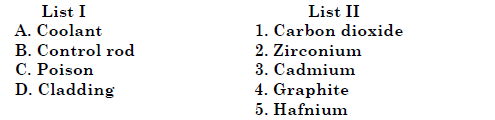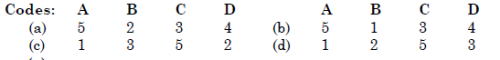Solution:
QUESTION: 10

Match List I (Material) With List II (Application) and select the correct answer.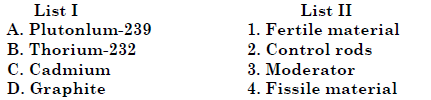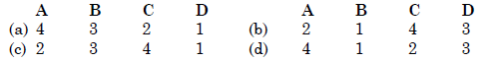Solution:
QUESTION: 11

Which one of the following is correct ? A nuclear reactor is said to be critical when the neutron population in the reactor core is

Solution:
QUESTION: 12

Assertion (A): Pressurized water reactor (PWR) nuclear power plants use superheated steam.

Reason (R): An increase in the superheat at constant pressure increases the cycle efficiency.

Solution:

Pressurized water reactor (PWR) nuclear power plants doesn’t use
superheated steam.

QUESTION: 13

Assertion (A): The thermal efficiency of a nuclear power plant using a boiling water reactor is higher than of a plant using a pressurized water reactor.

Reason (R): In a boiling water reactor, steam is directly allowed to be generated inthe reactor itself, whereas in a pressurized water reactor, steam is generated in aseparate boiler by heat exchanger device using water of the primary circuit which absorbs the fission energy.

Solution:

Both A and R are individually true but R is not the correct explanation of A. Because low pressure and temperature in secondary loop results in poor thermodynamic efficiency in PWR.

QUESTION: 14

Match List I with List II and select the correct answer using the codes given below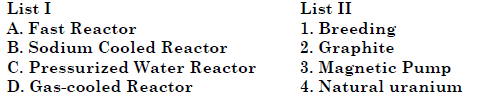the lists: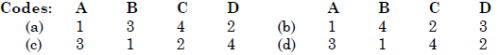Solution:

Since fast reactor is related with breeding, magnetic pump is needed for sodium cooled reactor, mainly natural uranium (with some enriched uranium) is used in pressurized water reactor, and graphite is moderator for gas cooled reactor.

QUESTION: 15

Assertion (A): A breeder reactor does not require moderator.

Reason (R): The parasite absorption of neutrons is low.

Solution:
QUESTION: 16

Enriched uranium is required as fuel in a nuclear reactor, if light water isused as moderator and coolant, because light water has

Solution:
QUESTION: 17

Which one of the following statements is correct ?

The mass defect is

Solution:
QUESTION: 18

Which one of the following pairs is not correctly matched?

Solution:

U-233 is not a fertile material.

QUESTION: 19

Uranium 238 is represented as 92U238 . What does it imply?

Solution: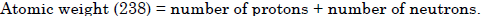QUESTION: 20

The energy released during the fission of one atom of Uranium-235 inmillion electron volts is about

Solution:

Energy released during fission of U-235 is 200 million electron volt